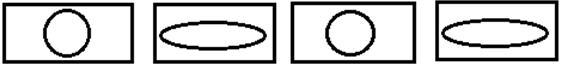Courses

Test: Number - 1

10 Questions MCQ Test Mathematics for Class 1: NCERT | Test: Number - 1

Description
Attempt Test: Number - 1 | 10 questions in 20 minutes | Mock test for Class 1 preparation | Free important questions MCQ to study Mathematics for Class 1: NCERT for Class 1 Exam | Download free PDF with solutions
QUESTION: 1

Which sign shows that a number is equal to other numbers

Solution:

Equals to (=) sign is used to show that two numbers are equal to each other

like 2 = 2.

QUESTION: 2

Solution:
QUESTION: 3

Which number is one less than 8 tens 4 ones?

Solution:
QUESTION: 4

Identify the odd one out.

Solution:
QUESTION: 5

Complete the following series.

Rs.11, Rs.12, Rs.13, Rs.14?

Solution:
QUESTION: 6

What is 7 tens and 2 ones?

Solution:
QUESTION: 7

Which of the following is the odd one in this series?

10, 30, 50, 70, 75, 90

Solution:
QUESTION: 8

Identify the next possible pattern and complete the series.Solution:
QUESTION: 9

Which of the following is the correct number name for 53?

Solution:
QUESTION: 10

Identify the odd one out in this series.

11, 12, 13, 14, 17

Solution:Use Code STAYHOME200 and get INR 200 additional OFF Use Coupon Code

Track your progress, build streaks, highlight & save important lessons and more!

Similar ContentRelated tests